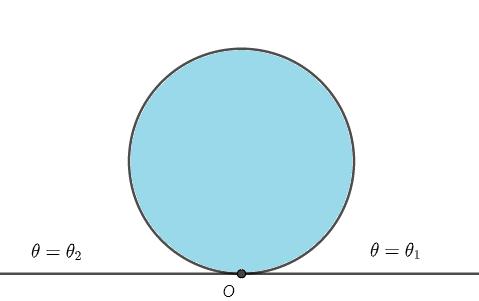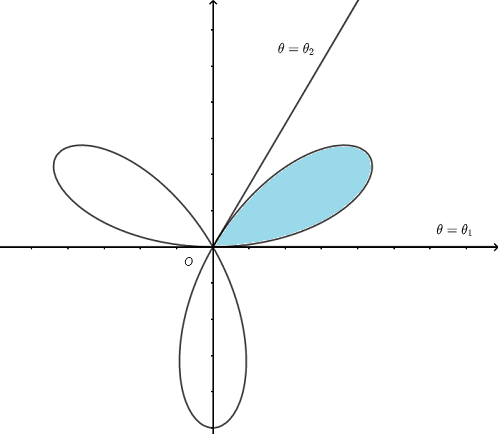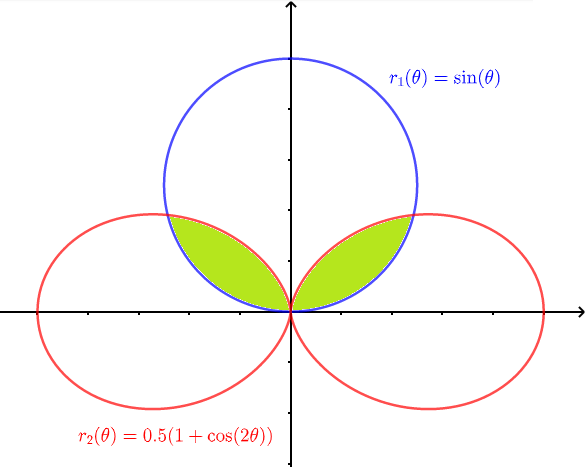# Calculate Area in Polar Coordinates

## Formula of Area in Polar Coordinates

 

The area bounded by a curve of polar equation $r(\theta)$ and by the rays $\theta = \theta_1$ and $\theta = \theta_2$ is given by the formula    $\dfrac{1}{2}\int_{\theta_1}^{\theta_2} \; r^2(\theta) \; d\theta$

## Examples and Solutions

Example 1
Use the formula given above to find the area of the circle enclosed by the curve $r(\theta) = 2 \sin (\theta)$ whose graph is shown below and compare the result to the formula of the area of a circle given by $\pi r^2$ where $r$ is the radius..Fig.2 - Circle in Polar Coordinates $r ( \theta) = 2 \sin \theta$

Solution to Example 1
Note that the circle is swept by the rays $\theta = \theta_1$ and $\theta = \theta_2$ and we need to find $\theta_1$ and $\theta_2$.Fig.3 - Circle Bounded by $r(\theta) = 2 \sin (\theta)$ Swept by the Rays $\theta = \theta_1$ and $\theta = \theta_2$
The circle may be assumed to start at the origin such that $r(\theta) = 0$ and finish at the origin $r(\theta) =0$. Hence the angles $\theta_1$ and $\theta_2$ are found by solving $r(\theta) = 0$. Which gives the equation
$\sin ( \theta) = 0$
which gives the following solutions
The solutions are: $\theta_1 = 0$ , $\theta_2 = \pi$
The area $A$ of the circle is given by
$A = \dfrac{1}{2}\int_{0}^{\pi} \; (2 \sin \theta )^2 \; d\theta$
Simplify

$A = 2\int_{0}^{\pi} \; (\sin \theta )^2 \; d\theta$

Use the trigonometric identity $\sin^2 \theta = \dfrac{1}{2} (1 - cos(2\theta) )$ and simplify to obtain
$A = \int_{0}^{\pi} \; (1 - cos(2\theta) ) \; d\theta$
Evaluate the integral
$A = \int_{0}^{\pi} \; \left[\theta -\dfrac{1}{2}\sin (2 \theta ) \right]_0^{\pi} = \pi$
The area of the given circle whose radius is $1$ could have been calculated using the formula $\pi \cdot r^2 = \pi \cdot 1^2 = \pi$

Example 2
Find the area of the region enclosed by the curve $r(\theta) = \sin ( 3 \theta)$ whose graph is shown below.Fig.4 -Plot of Curve $r (\theta) = \sin (3 \theta)$

Solution to Example 2
Because of the symmetry of the graph of the curve, we need to find the area enclosed by one loop and then multiply the result by $3$.Fig.5 - Pplot of Curve $r (\theta) = \sin (3 \theta)$ with Limits of Integration
The loop on the right (in blue) starts at the origin $r (\theta) = 0$ and finish at the origin $r (\theta) = 0$. Hence $\theta_1$ and $\theta_2$ are found by solving $r(\theta) = 0$. Which gives the equation
$\sin (3 \theta) = 0$
which gives the general solutions: $3 \theta = n \pi$ with $n = 0, \pm 1, \pm 2, ...$
We need the solutions in quadrant (I) which are given by
$\theta_1 = 0$ and $\theta_2 = \dfrac{\pi}{3}$
The area of the shaded (in blue) loop is given by
$A = \dfrac{1}{2} \int_{0}^{\frac{\pi}{3}} \; (\sin 3 \theta )^2 \; d\theta$
Use the trigonometric identity $\sin^2 (3\theta) = \dfrac{1}{2} (1 - cos(6\theta) )$ and simplify to obtain
$A = \dfrac{1}{4} \int_{0}^{\frac{\pi}{3}} \; (1 - cos(6\theta)) \; d\theta$
Evaluate the integral
$A = \dfrac{1}{4} \left[\theta - \frac{1}{6}\sin (6\theta) \right]_0^{\frac{\pi}{3}}= \dfrac{\pi}{12}$
The total are of all three loops is given by $3 A = \dfrac{ \pi}{4}$

Example 3
Find the area of the region enclosed by the curves $r_1(\theta) = \sin (\theta)$ and $r_2(\theta) = 0.5 (1+\cos ( 2\theta))$ as shown below.Fig.6 -Plot of the Curves $r_1 (\theta) = \sin (\theta)$ and $r_2(\theta) = 0.5 (1+\cos ( 2\theta))$

Solution to Example 3
Because of the symmetry, we need to find the area of the surface enclosed by the two curves on the right, as shown below, and multiply the result by two.Fig.7 - Plot of the Curves $r_1 (\theta) = \sin (\theta)$ and $r_3(\theta) = 0.5 (1+\cos ( 2\theta))$ with Limits of Integration
The area to be found is made up of two parts: $A_1$ and $A_2$ given by
$A_1 = \dfrac{1}{2}\int_{\theta_1}^{\theta_2} \; r_1^2(\theta) \; d\theta$ $A_2 = \dfrac{1}{2}\int_{\theta_2}^{\theta_3} \; r_2^2(\theta) \; d\theta$
Find the limits of integration $\theta_1$, $\theta_2$ and $\theta_3$.

a) $\theta_1$ is found by solving the equation $r_1 (\theta) = 0$ , hence $\sin (\theta) = 0$ which gives the general solution $\theta_1 = n \pi$ with $n = 0, \pm 1, \pm 2, ...$
The solution needed is $\theta_1 = 0$

b) $\theta_3$ is found by solving the equation $r_2 (\theta) = 0$ which gives $0.5 (1+\cos ( 2\theta)) = 0$
$\cos ( 2\theta) = -1$ which gives the general solutions: $\theta = n \dfrac{\pi}{2}$ with $n = 0, \pm 1, \pm 2, ...$
The needed solution $\theta_3 = \dfrac{\pi}{2}$

c) $\theta_2$ correspond to a ray that passes through the point of intersection of the curves $r_1(\theta)$ and $r_2(\theta)$ and is found by solving the equation $r_1(\theta) = r_2(\theta)$ writen as
$\sin (\theta) = 0.5 (1+\cos ( 2\theta))$
Use the identity $\cos ( 2\theta) = 1 - 2 \sin^2 \theta$, and rewrite the equation as
$\sin (\theta) = 0.5 (1+(1 - 2 \sin^2 \theta))$
Simplify
$\sin^2 \theta + \sin (\theta) - 1 = 0$
Solve the above quadratic like equation to obtain the solutions
$\sin (\theta')=\dfrac{-1+\sqrt{5}}{2} \approx 0.61803$ and $\quad \sin (\theta")=\dfrac{-1-\sqrt{5}}{2} \approx -1.61803$
The quation $\quad \sin (\theta") \approx -1.61803$ has no solution.
Hence the general solution in quadrant (I) is given by $\theta' = \arcsin (0.61803) + 2 n \pi$
which gives $\theta_2 = 0.61803$
$A_1$ and $A_2$ may be written as
$A_1 = \dfrac{1}{2}\int_{0}^{0.61803} \; \sin^2(\theta) \; d\theta$ $A_2 = \dfrac{1}{2}\int_{0.61803}^{\frac{\pi}{2}} \; (0.5 (1+\cos ( 2\theta)))^2 \; d\theta$ Use the identity $\sin^2 \theta = \dfrac{1}{2} (1 - cos(2\theta) )$ in $A_1$ and simplify $A_1 = \dfrac{1}{4}\int_{0}^{0.61803} \; (1 - cos(2\theta) ) \; d\theta$ Evaluate $A_1$ $A_1 = \dfrac{1}{4}[\theta -\dfrac{1}{2}\sin (2\theta) ]_{0}^{0.61803} \approx 0.03644625$

Expand the integrand in $A_2$
$A_2 = \dfrac{1}{2}\int_{0.61803}^{\frac{\pi}{2}} \; 0.5^2 (1+\cos^2( 2\theta)+2 \cos (2\theta)) \; d\theta$
Use the identity $\cos^2 (2\theta) = \dfrac{1}{2} (1 + cos(4\theta) )$ in $A_2$ and simplify
$A_2 = \dfrac{0.5^2}{2}\int_{0.61803}^{\frac{\pi}{2}} \; (1+ \dfrac{1}{2} (1 + cos(4\theta) ) + 2 \cos (2\theta)) \; d\theta$
Evaluate $A_2$
$\dfrac{0.5^2}{2} \left[ \frac{3}{2}\theta+\frac{1}{8}\sin (4 \theta )+\sin (2 \theta) \right]_{0.61803}^{\frac{\pi}{2}} \approx 0.050885$
The total area $A$ enclosed by the two curves is $2 (A_1 + A_2)$
$A \approx 0.1746625$